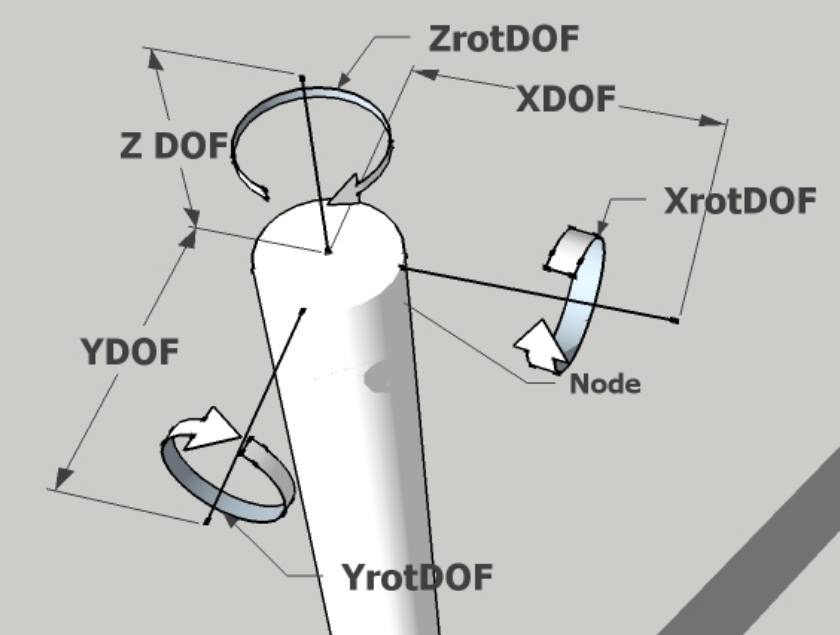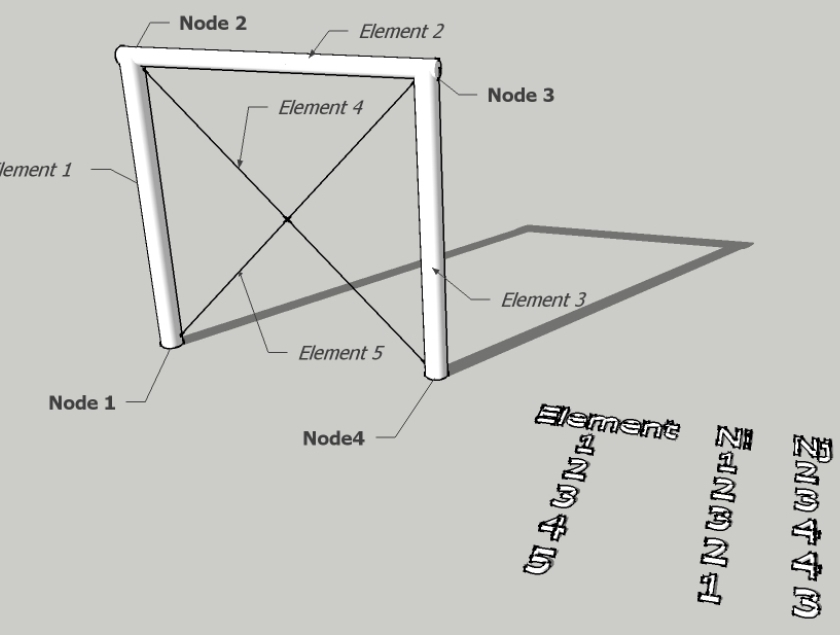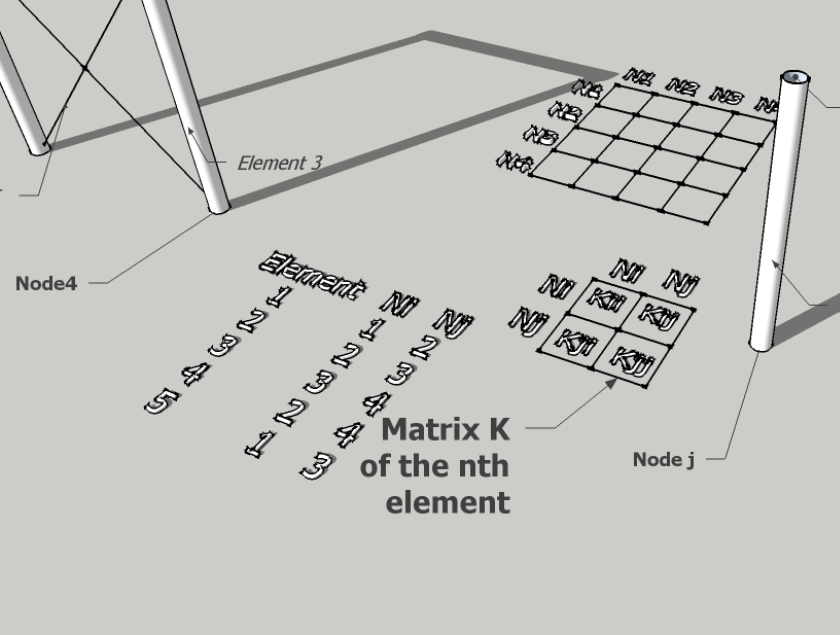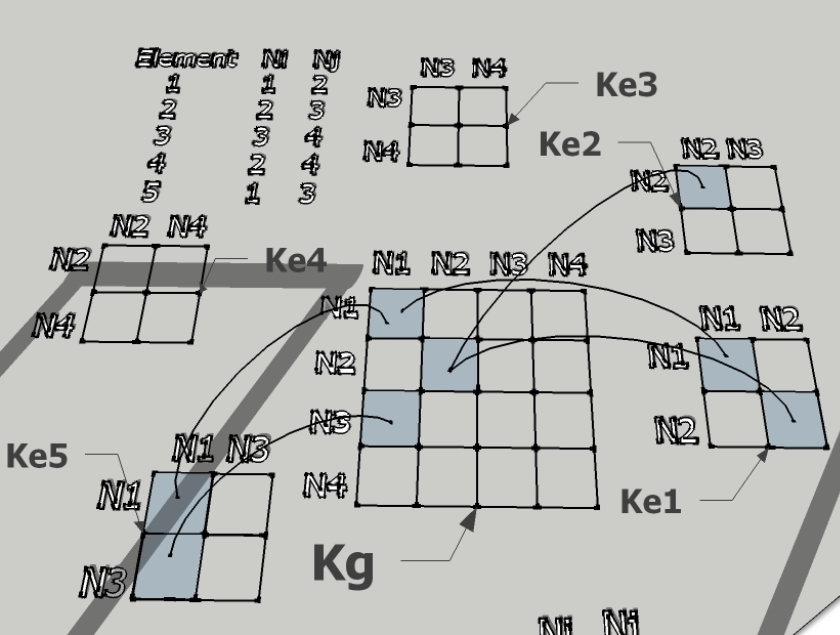FEA (Finite Element Analysis)

FEA, or finite element analysis, is a technique for predicting the response of structures and materials to environmental factors such as forces, heat and vibration. FEA is a fairly recent discipline that provides the numerical solution of governing physical equations over complicated geometric domains.  As the name suggests the part/component under analysis is divided into several small regions called ‘finite elements’.

• FEA Steps:
• Preprocessing
¨ Solid Modeling
¨ Meshing
¨ Boundary Conditions
• Processing / Solving
• Post processing
This involves interpretation of results